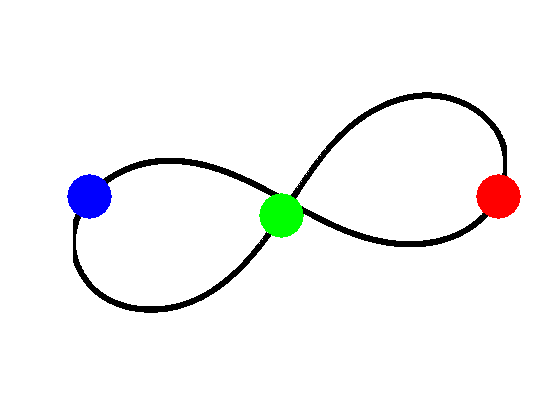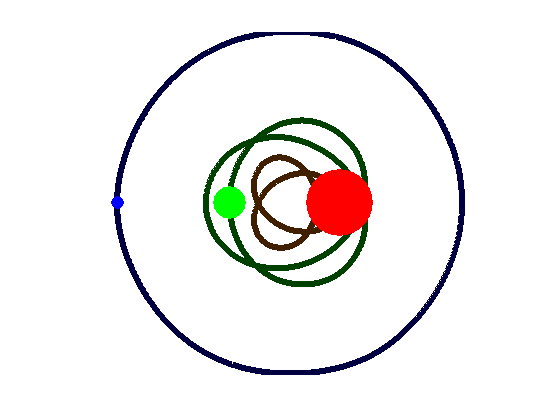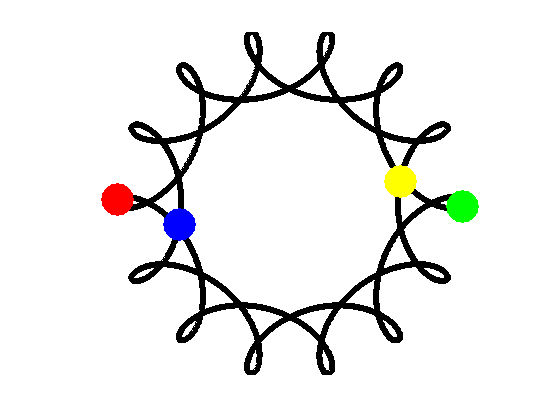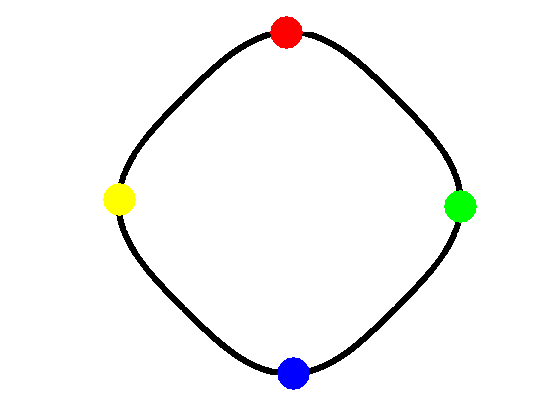# n-body problem

In the n-body problem, we seek to describe the interaction between a fixed number of objects under Newton’s Law of Gravity. A simple equation given by Newton in 1687 describes the gravitational attraction between two objects. Using a computer, we can compute the sum of the forces acting on a fixed number of objects. Once this is known, we can deduce where the object will be in the next time step.

These images show some newly discovered periodic (repeating) orbits for 3 and 4 bodies.Caption A: Three bodies with equal mass orbiting on a figure eight. This result was published in 2000.Caption B: Three bodies of unequal mass. This system is similar to a planet orbiting a binary star system.Caption C: Two pairs of bodies orbiting about each other.Caption D: An orbit discovered in 2008 by Tiancheng Ouyang, Duokui Yan, and Skyler Simmons at BYU.

This problem is far from a mathematical curiosity. For example, NASA uses computations like these to model motions for launches of space probes and other spacecraft.